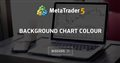# Hey coders! Is it possible to change background color of the chart when signal comes.? for MT5

when signal arrow appear on the chart i want to change chart background color, suggest me how to do it.....

```//+------------------------------------------------------------------+
//|                                       Indicator: stock-arrow.mq5 |
//+------------------------------------------------------------------+
#property version   "1.00"
#property description ""

//--- indicator settings
#property indicator_chart_window
#property indicator_buffers 2
#property indicator_plots 2

#property indicator_type1 DRAW_ARROW
#property indicator_width1 5
#property indicator_color1 Green

#property indicator_type2 DRAW_ARROW
#property indicator_width2 5
#property indicator_color2 Red
#property indicator_label2 "Sell"

//--- indicator buffers
double Buffer1[];
double Buffer2[];

bool Send_Email = false;
double myPoint; //initialized in OnInit
int Stochastic_handle;
double Stochastic_Main[];
double Stochastic_Signal[];
double Low[];
double High[];

{
if(type == "print")
Print(message);
else if(type == "error")
{
Print(type+" | stock-arrow @ "+Symbol()+","+IntegerToString(Period())+" | "+message);
}
else if(type == "order")
{
}
else if(type == "modify")
{
}
else if(type == "indicator")
{
Print(type+" | stock-arrow @ "+Symbol()+","+IntegerToString(Period())+" | "+message);
if(Send_Email) SendMail("stock-arrow", type+" | stock-arrow @ "+Symbol()+","+IntegerToString(Period())+" | "+message);
}
}

//+------------------------------------------------------------------+
//| Custom indicator initialization function                         |
//+------------------------------------------------------------------+
int OnInit()
{
SetIndexBuffer(0, Buffer1);
PlotIndexSetDouble(0, PLOT_EMPTY_VALUE, EMPTY_VALUE);
PlotIndexSetInteger(0, PLOT_ARROW, 233); PlotIndexSetInteger(0,PLOT_ARROW_SHIFT, 20);
SetIndexBuffer(1, Buffer2);
PlotIndexSetDouble(1, PLOT_EMPTY_VALUE, EMPTY_VALUE);
PlotIndexSetInteger(1, PLOT_ARROW, 234); PlotIndexSetInteger(1,PLOT_ARROW_SHIFT, -20);
//initialize myPoint
myPoint = Point();
if(Digits() == 5 || Digits() == 3)
{
myPoint *= 10;
}
Stochastic_handle = iStochastic(NULL, PERIOD_CURRENT, 5, 3, 3, MODE_SMA, STO_LOWHIGH);
if(Stochastic_handle < 0)
{
Print("The creation of iStochastic has failed: Stochastic_handle=", INVALID_HANDLE);
Print("Runtime error = ", GetLastError());
return(INIT_FAILED);
}

return(INIT_SUCCEEDED);
}

//+------------------------------------------------------------------+
//| Custom indicator iteration function                              |
//+------------------------------------------------------------------+
int OnCalculate(const int rates_total,
const int prev_calculated,
const datetime& time[],
const double& open[],
const double& high[],
const double& low[],
const double& close[],
const long& tick_volume[],
const long& volume[],
{
int limit = rates_total - prev_calculated;
//--- counting from 0 to rates_total
ArraySetAsSeries(Buffer1, true);
ArraySetAsSeries(Buffer2, true);
//--- initial zero
if(prev_calculated < 1)
{
ArrayInitialize(Buffer1, EMPTY_VALUE);
ArrayInitialize(Buffer2, EMPTY_VALUE);
}
else
limit++;
datetime Time[];

if(BarsCalculated(Stochastic_handle) <= 0)
return(0);
if(CopyBuffer(Stochastic_handle, MAIN_LINE, 0, rates_total, Stochastic_Main) <= 0) return(rates_total);
ArraySetAsSeries(Stochastic_Main, true);
if(BarsCalculated(Stochastic_handle) <= 0)
return(0);
if(CopyBuffer(Stochastic_handle, SIGNAL_LINE, 0, rates_total, Stochastic_Signal) <= 0) return(rates_total);
ArraySetAsSeries(Stochastic_Signal, true);
if(CopyLow(Symbol(), PERIOD_CURRENT, 0, rates_total, Low) <= 0) return(rates_total);
ArraySetAsSeries(Low, true);
if(CopyHigh(Symbol(), PERIOD_CURRENT, 0, rates_total, High) <= 0) return(rates_total);
ArraySetAsSeries(High, true);
if(CopyTime(Symbol(), Period(), 0, rates_total, Time) <= 0) return(rates_total);
ArraySetAsSeries(Time, true);
//--- main loop
for(int i = limit-1; i >= 0; i--)
{
if (i >= MathMin(5000-1, rates_total-1-50)) continue; //omit some old rates to prevent "Array out of range" or slow calculation

//Indicator Buffer 1
if(Stochastic_Main[i] > Stochastic_Signal[i]
&& Stochastic_Main[i+1] < Stochastic_Signal[i+1] //Stochastic Oscillator crosses above Stochastic Oscillator
)
{
Buffer1[i] = Low[i]; //Set indicator value at Candlestick Low
}
else
{
Buffer1[i] = EMPTY_VALUE;
}
//Indicator Buffer 2
if(Stochastic_Main[i] < Stochastic_Signal[i]
&& Stochastic_Main[i+1] > Stochastic_Signal[i+1] //Stochastic Oscillator crosses below Stochastic Oscillator
)
{
Buffer2[i] = High[i]; //Set indicator value at Candlestick High
}
else
{
Buffer2[i] = EMPTY_VALUE;
}
}
return(rates_total);
}
//+------------------------------------------------------------------+```Background Chart Colour
• 2014.09.26
• www.mql5.com
Hi I have the attached indicator, but have been unable to get hold of the clever programmer...

I doubts,he asking chart background color changing according to arrows (up/down) an selectable color choice when arrow up and different coloring choice when arrow to down

mntiwana #:

I doubts,he asking chart background color changing according to arrows (up/down) an selectable color choice when arrow up and different coloring choice when arrow to down

Exactly, i think you understand, what i want, please suggest me, how to do that.

I don't see why not

Global variable:

`input color colorChangeOnSignal = clrBlack;`

In OnCalculate:

```if(signal){

ChartSetInteger(0, CHART_COLOR_BACKGROUND, colorChangeOnSignal);
}```

phade #: if(signal){   ChartSetInteger(0, CHART_COLOR_BACKGROUND, colorChangeOnSignal); }

maxtitu835 #: please add it on attached code, iam newbie on coding.
• Usually people who can't code don't receive free help on this forum.
• If you show your attempts and describe your problem clearly, you will most probably receive an answer from the community. Use the CODE button (Alt-S) when inserting code.
• To learn MQL programming, you can research the many available Articles on the subject, or examples in the Codebase, as well as reference the online Documentation.
• If you do not want to learn to code, that is not a problem. You can either look at the Codebase if something free already exists, or in the Market for paid products (also sometimes free). However, recommendations or suggestions for Market products are not allowed on the forum, so you will have to do your own research.
• Finally, you also have the option to hire a programmer in the Freelance section.

maxtitu835 #:

But you didn't say what the signal should be.

If the indicator should change background when the Alert occurs...for example...then it would be as such:

```// global variables at the top of the script
input bool bgChangerActive = true;
input color colorChangeOnSignal = clrRed;```
```  for(int i = limit-1; i >= 0; i--)
{
if (i >= MathMin(5000-1, rates_total-1-50)) continue; //omit some old rates to prevent "Array out of range" or slow calculation

//Indicator Buffer 1
if(Stochastic_Main[i] > Stochastic_Signal[i] && Stochastic_Main[i+1] < Stochastic_Signal[i+1]) //Stochastic Oscillator crosses above Stochastic Oscillator
{
if(bgChangerActive){
ChartSetInteger(0, CHART_COLOR_BACKGROUND, colorChangeOnSignal);
}
Buffer1[i] = Low[i]; //Set indicator value at Candlestick Low
}
else
{
Buffer1[i] = EMPTY_VALUE;
}
//Indicator Buffer 2
if(Stochastic_Main[i] < Stochastic_Signal[i] && Stochastic_Main[i+1] > Stochastic_Signal[i+1]) //Stochastic Oscillator crosses below Stochastic Oscillator
{
Buffer2[i] = High[i]; //Set indicator value at Candlestick High
}
else
{
Buffer2[i] = EMPTY_VALUE;
}
}```